Math Central - mathcentral.uregina.caProblem of the MonthCurrentproblem Recent problemswith solutions
 Older problems from 2005/2006 06/07 07/08 08/09 09/10 10/11 11/12

Solution to November 2006 Problem

The problem:
We start with two positive real numbers a0 and a1, and obtain an infinite sequence whose terms satisfy

an+1 an-1 = an + 1.

Show that an+ 5 = an for all n.

 Saïd Amghibech (Québec) Wolfgang Kais (Germany) Mario Antunez (Honduras) Normand LaLiberté (Ontario) Ricardo Barroso Campos (Spain) Matthew Lim (USA) Pierre Bornsztein (France) Juan Mir Pieras (Spain) Bernard Carpentier (France) Nikhil V. Nair (India) K.A. Chandrashekara (India) Wilfrid Pillard (France) Stefaan De Winter (Belgium) Mark Pilloff (USA) Sébastien Dumortier (France) Manoranjan Sahu (India) Philippe Fondanaiche (France) Shiv Mohan Sharma (internet) Baptiste Gorin (France) A. Teitelmam (Israel) Xavier Hecquet (France) Zac Friggstad (Alberta)

We received a variety of solutions, but most correspondents were satisfied using a straightforward argument.  We present such a solution first, followed by two simplified versions.  The sequence itself is related to some ideas of Gauss; we will conclude with his treatment of the problem, including his proof by deus ex machina.

The straightforward solution.  Since we need only six letters, let us replace six successive elements an, ... , an+5 of the sequence by the letters in order from u to z.  In this notation we are given the four equations

(1) uw = v+1,     (2) vx = w+1,     (3) wy = x+1,     (4) xz = y+1,

and are to prove that z = u.

From (1) we get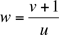.
Plug this value of w into (2), and continue in this way to get successively,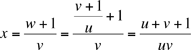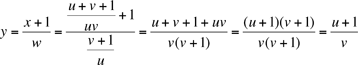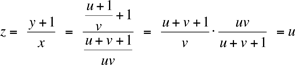Thus we have z = u, so that an+5 = an for any n, as desired.  Since all terms are positive, we never have to worry about dividing by zero, so that each step is valid.  As Mir points out, we can relax the positivity condition by simply requiring that a0 + 1 ≠ 0 , a1 + 1 ≠ 0, and a0 + a1 + 1 ≠ 0.

A shortcut from Saïd Amghibech (that we modified somewhat).
We use the same notation as in the first solution.  Multiply equation (4) by w to get

wxz =(4) wy + w =(3) x + 1 + w =(2) x + xv = x(1 + v) =(1) xuw

Cancel the wx from both sides to conclude that z = u.
We can find another easy solution by noticing that the given condition is symmetric in n+1 and n-1; in other words, the formula for increasing n is the same as for decreasing n.  That suggests we start in the middle, letting an = w and an+1 = x.  We then have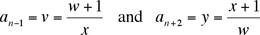from which we get (as in the first solution)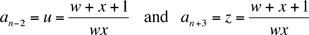Thus, we get an-2 = an+3 for all n, which is another way of saying that the sequence is a 5-cycle.

A solution from Gauss.
In truth, Gauss did not send us his solution.  We got it from H.S.M. Coxeter in his article, "Frieze Patterns", Acta Arithmetica 26 (1971), pages 297-310.  See pages 297 and 298.  In Coxeter's words,
The story begins in 1602, when Nathaniel Torporley (1564-1632) began to investigate the five "parts" a, A, b, B, c of a right-angled spherical triangle (right-angled at C).  According to De Morgan, Torporley anticipated by a dozen years the famous rules of Napier which Gauss embodied in his pentagramma mirificum.  [Napier's rules allows one to derive a large portion of spherical trigonometry with very little effort; see, for example, what the Wikipedia has to say about Napier and his rules.]  Gauss used the identity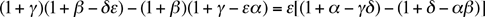to prove that any three of the four relations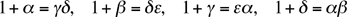implies the remaining one and also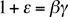The pentagramma mirificum is a spherical pentagram formed by five successively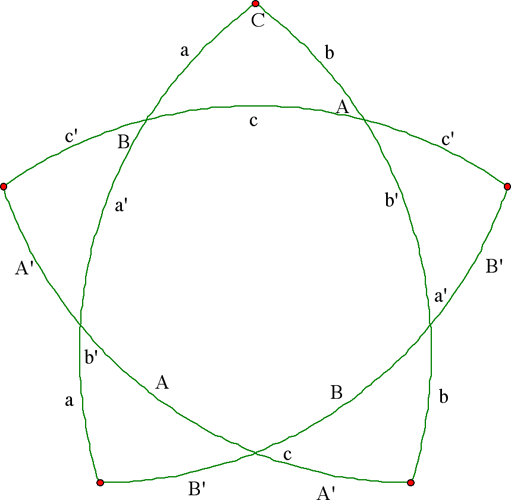orthogonal great circle arcs.  The "core" of the pentagram is a pentagon whose vertices are (obviously) poles of these five arcs; it is thus a self-polar pentagon.  [This means that if a vertex of the inside pentagon is placed at the north pole of the sphere, the opposite side lies along the equator.]  The whole figure can be derived from the right-angled triangle ABC (appearing at the top) by extending the sides and drawing also the polar great circles of the vertices A and B.  Using a prime to indicate the complement

x' = 1/2 π - x  ,

we easily see that the sides of the self-polar pentagon are

A, B, b', c, a'

while the remaining arcs and angles are as indicated in the figure.  Clearly, any equation connecting the five "parts" (thus amended) remains valid when they are cyclically permuted.  This is Napier's observation (except that he used the alternated cycle a', B, c, A, b').

Gauss defined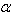= tan2 A,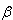= tan2 B,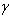= tan2 b',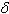= tan2 c,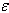= tan2 a'
and used one of the classical relations (such as cos c = cot A cot B) to derive
sec2 A =,                sec2 B =,          sec2 b' =,
sec2 c =,                 sec2 a' =.
Note that these assignments imply that 1 +=, and so on.  Coxeter provides further explanation and applications.  In particular, he applies the sequence to provide the basis for trigonometry in the hyperbolic plane, but we will stop the story here.

 Math Central is supported by the University of Regina and the Pacific Institute for the Mathematical Sciences.about math central :: site map :: links :: notre site français